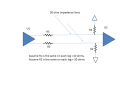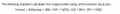# Termination for Differential Pair - 50 ohm transmission line

#### matthej

Joined Oct 10, 2020
58
Hi,

I have a circuit (see attached) and I am wondering what happens to a differential signal that gets generated in U1 and received by U2.

Lets say the signal has a 400-600mv swing at the output of U1.

Would I see the following at U2? Vout = R2/Rs+R2 * Vin : Vout= 50/10+50 * 600mV = 500mV

OR

Vout = R2/Rs+R2+50 ohms (transmission line): Vout = 50/10+50+50*600mV = 272mVIn other words, do you factor in the 50 ohm trace?

Thanks!

#### crutschow

Joined Mar 14, 2008
28,206
Neither of you equations gives the values you calculated.

Hint: An ideal (lossless) 50Ω transmission line driving a 50Ω load has an output voltage at the load equal to the voltage at the driving end of the line.

#### Deleted member 115935

Joined Dec 31, 1969
0
Hi,

I have a circuit (see attached) and I am wondering what happens to a differential signal that gets generated in U1 and received by U2.

Lets say the signal has a 400-600mv swing at the output of U1.

Would I see the following at U2? Vout = R2/Rs+R2 * Vin : Vout= 50/10+50 * 600mV = 500mV

OR

Vout = R2/Rs+R2+50 ohms (transmission line): Vout = 50/10+50+50*600mV = 272mV

View attachment 242372
In other words, do you factor in the 50 ohm trace?

Thanks!
So, this is a differential line,
50 ohms differential impedance is very low, it would nomaly be 100 ohms differential,
At the receiver, you show two resistors to ground, this would in practice kill the differential
There should be 100 ohms between the receiver pairs, no termination to ground,,

Then the receiver is receiving the differential between the two traces, no reference to ground
hence its name,

You will also see , that although the transmitter then will produce voltages with reference to its ground,
its the difference in the two outputs that matter at the receiver Assuming a perfect transmitter, line and receiver, the receiver subtracts one input from its other. The Transmitter has the same noise on both its outputs, and the transmission line has the same noise induced on both its wires, so at the perfect receiver, subtracting removes the noise.

Also , you would "never" put 10 ohm series resistors on differential lines,
as they are never going to be the exact same, they are going to put a dc offset onto the differential voltage.

It does look more like you have taken a pair of 50 ohm single ended traces,
and tried to use two as a differential, which is not the way to go,

#### matthej

Joined Oct 10, 2020
58
Last edited:

#### Deleted member 115935

Joined Dec 31, 1969
0
No,
A 50 ohm trace does NOT have a resistance of 50 OHMs,
its a single ended impedance.

If you measured it , you would see zero ohms.

The Rp and Rn, are Thevlin equivilent resistors to Vt, not to ground as you showed.
Vt , is a nominal mid rail voltage, more explicitly the bias voltage the receiver needs.

#### matthej

Joined Oct 10, 2020
58
No,
A 50 ohm trace does NOT have a resistance of 50 OHMs,
its a single ended impedance.

If you measured it , you would see zero ohms.

The Rp and Rn, are Thevlin equivilent resistors to Vt, not to ground as you showed.
Vt , is a nominal mid rail voltage, more explicitly the bias voltage the receiver needs.

I see a 50 in the equation for Vswing : Where is this 50 coming from (and apologies for now showing two diagrams)#### Deleted member 115935

Joined Dec 31, 1969
0

#### matthej

Joined Oct 10, 2020
58
And now combine to get an equation for the differential at the receiver
But you are factoring in the trace impendence into this equation, correct?

#### Deleted member 115935

Joined Dec 31, 1969
0
Did you try the combination,
and work on premise that the two traces are the same impedance ?

we have now had two diagrams, and muliple randon equations,
where is this comming from ?

They look like cuts form books,
taken out of context.

Can we have a background please,

one other thing might be worth adding,

• Differential impedance is the impedance measured between two conductors when they are driven in the differential mode.

Its not a resistance,

Last edited by a moderator:

#### Deleted member 115935

Joined Dec 31, 1969
0
But you are factoring in the trace impendence into this equation, correct?

Do you get single ended and differential impedance ?

An example came to mind.

If I had a pair of 50 ohm resistors and connected them in series, I would have 100 ohms.

If I had a length of cable with 50 ohms single ended impedance,
and doubled the length, the single ended impedance would still be 50 ohms,.

If the cable had a resistance of say 1 ohm, and I doubled it , the resistance would now be 2 ohms, as expected, but the single ended impedance would still be 50 ohms.

confusing ah what !!